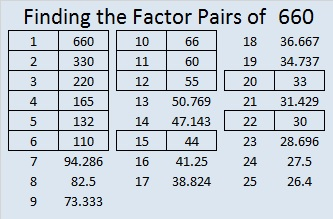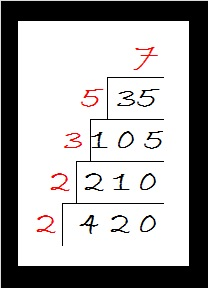# 660 My Two-Year Blogiversary

Today is my 2 year blogiversary and my 660th post. Thank you to all my readers and followers. I really appreciate all of you.

What better way is there to celebrate than with a gorgeous cake? Enjoy!

The cake method of finding prime factors makes a beautiful cake for the number 660 because 660 has several prime factors and the largest one, 11, looks like a couple of candles to top it off perfectly.660 has a lot of factors, 24 in fact. 660 is a special number for several reasons:

No number less than 660 has more factors than it does, but 360, 420, 480, 504, 540, 600, and 630 each have just as many.

660 has so many factors that it seems natural for it to between twin primes, 659 and 661.

660 is the hypotenuse of the Pythagorean triple 396-528-660. What is the greatest common factor of those three numbers?

660 is the sum of consecutive prime numbers three different ways. Prime number 101 is in two of those ways:

• 157 + 163 + 167 + 173 = 660
• 101 + 103 + 107 + 109 + 113 + 127 = 660
• 67 + 71 + 73 + 79 + 83 + 89 + 97 + 101 = 660

—————————————————————————————————

• 660 is a composite number.
• Prime factorization: 660 = 2 x 2 x 3 x 5 x 11, which can be written 660 = 2² x 3 x 5 x 11
• The exponents in the prime factorization are 2, 1, 1, and 1. Adding one to each and multiplying we get (2 + 1)(1 + 1)(1 + 1)(1 + 1) = 3 x 2 x 2 x 2 = 24. Therefore 660 has exactly 24 factors.
• Factors of 660: 1, 2, 3, 4, 5, 6, 10, 11, 12, 15, 20, 22, 30, 33, 44, 55, 60, 66, 110, 132, 165, 220, 330, 660
• Factor pairs: 660 = 1 x 660, 2 x 330, 3 x 220, 4 x 165, 5 x 132, 6 x 110, 10 x 66, 11 x 60, 12 x 55, 15 x 44, 20 x 33, or 22 x 30
• Taking the factor pair with the largest square number factor, we get √660 = (√4)(√165) = 2√165 ≈ 25.690465.—————————————————————————————————

# 420 Factor Trees

Did you know that the sum of all the prime numbers between 100 and 110 equals 420? Yes, 101 + 103 + 107 + 109 = 420.

Since 20 × 21 = 420, we know that 420 is the sum of the first 20 EVEN numbers. Thus,

• 2 + 4 + 6 + 8 + 10 + 12 + 14 + 16 + 18 + 20 + 22 + 24 + 26 + 28 + 30 + 32 + 34 + 36 + 38 + 40 = 420.

420 is the smallest number that can be divided evenly by all the natural numbers from 1 to 7.

420 has a LOT of factors, more than most people would think it does.  In fact, of all of the numbers from 1 to 420, there is only one number, 360, that has as many factors as 420 has.

There are 4 different prime numbers that can divide evenly into 420. Here are those factor trees:Many, but not all, of the factors of 420 are listed somewhere on those four trees.

• 420 is a composite number.
• Prime factorization: 420 = 2 x 2 x 3 x 5 x 7, which can be written 420 = (2^2) x 3 x 5 x 7
• The exponents in the prime factorization are 2, 1, 1, and 1. Adding one to each and multiplying we get (2 + 1)(1 + 1)(1 + 1)(1 + 1) = 3 x 2 x 2 x 2 = 24. Therefore 420 has exactly 24 factors.
• Factors of 420: 1, 2, 3, 4, 5, 6, 7, 10, 12, 14, 15, 20, 21, 28, 30, 35, 42, 60, 70, 84, 105, 140, 210, 420
• Factor pairs: 420 = 1 x 420, 2 x 210, 3 x 140, 4 x 105, 5 x 84, 6 x 70, 7 x 60, 10 x 42, 12 x 35, 14 x 30, 15 x 28, or 20 x 21
• Taking the factor pair with the largest square number factor, we get √420 = (√4)(√105) = 2√105 ≈ 20.4939Each factor pair, except 1 x 420, can make its own factor tree. Here are some factor trees featuring the other seven factor pairs:If I hadn’t made all the prime numbers in red, it’s possible that one or more of the prime numbers might get forgotten. That is why I prefer the cake method for finding the prime factorization of a number. All of the prime numbers are listed in numerical order on the outside of the cake.